## Autograd and the derivative of an integral function

| categories: | tags: | View Comments

There are many functions that are defined by integrals. The error function, for example is defined by $$erf(x) = \frac{2}{\sqrt{\pi}}\int_0^x e^{-t^2}dt$$.

Another example is:

$$\phi(\alpha) = \int_0^1 \frac{\alpha}{x^2 + \alpha^2} dx$$.

We have reasonable ways to evaluate these functions numerically, e.g. scipy.integrate.quad, or numpy.trapz, but what about the derivatives of these functions? The analytical way to do this is to use the Leibniz rule, which involves integrating a derivative and evaluating it at the limits. For some functions, this may also lead to new integrals you have to numerically evaluate. Today, we consider the role that automatic differentiation can play in this.

The idea is simple, we define a function in Python as usual, and in the function body calculate the integral in a program. Then we use autograd to get the derivative of the function.

In this case, we have an analytical derivative to compare the answers to:

$$\frac{d\phi}{d\alpha} = -\frac{1}{1 + \alpha^2}$$.

## 1 Example 1

For simplicity, I am going to approximate the integral with the trapezoid method in vectorized form. Here is our program to define $$\phi(\alpha)$$. I found we need a pretty dense grid on the x value so that we have a pretty accurate integral, especially near $$x=0$$ where there is a singularity as α goes to zero. That doesn't worry me too much, there are better integral approximations to use, including Simpson's method, adaptive methods and perhaps quadrature. If you define them so autograd can use them, they should all work. I chose the trapezoidal method because it is simple to implement here. Note, however, the autograd.numpy wrappers don't have a definition for numpy.trapz to use it directly. You could add one, or just do this.

import autograd.numpy as np

def trapz(y, x):
d = np.diff(x)
return np.sum((y[0:-1] + y[1:]) * d / 2)

def phi(alpha):
x = np.linspace(0, 1, 1000)
y = alpha / (x**2 + alpha**2)
return trapz(y, x)

# This is the derivative here!


Now, we can plot the derivatives. I will plot both the analytical and automatic differentiated results.

%matplotlib inline
import matplotlib.pyplot as plt

alpha = np.linspace(0.01, 1)

# The AD derivative function is not vectorized, so we use this list comprehension.
dphidalpha = [adphi(a) for a in alpha]

def analytical_dphi(alpha):
return -1 / (1 + alpha**2)

plt.plot(alpha, analytical_dphi(alpha), label='analytical')
plt.xlabel(r'$\alpha$')
plt.ylabel(r'$frac{d\phi}{d\alpha}$')
plt.legend()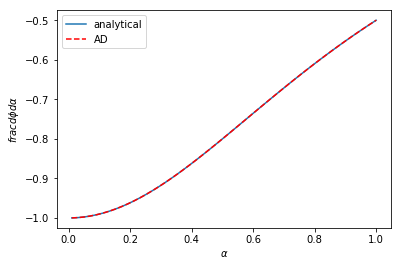Visually, these are indistinguishable from each other. We can look at the errors too, and here we see they are negligible, and probably we can attribute them to the approximation we use for the integral, and not due to automatic differentiation.

perr = (analytical_dphi(alpha) - dphidalpha) / analytical_dphi(alpha) * 100
plt.plot(alpha, perr, label='analytical')
plt.xlabel(r'$\alpha$')
plt.ylabel('%error')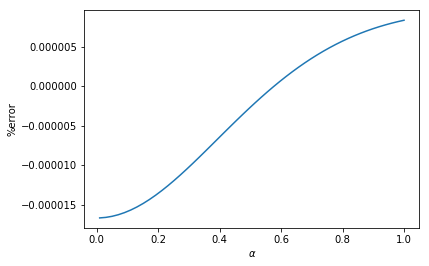## 2 Example 2

In example 2 there is this function, which has variable limits:

$$f(x) = \int_{\sin x}^{\cos x} \cosh t^2 dt$$

What is $$f'(x)$$ here? It can be derived with some effort and it is:

$$f'(x) = -\cosh(\cos^2 x) \sin x - \cosh(\sin^2 x) \cos x$$

This function was kind of fun to code up, I hadn't thought about how to represent variable limits, but here it is.

def f(x):
a = np.sin(x)
b = np.cos(x)
t = np.linspace(a, b, 1000)
y = np.cosh(t**2)
return trapz(y, t)

# Here is our derivative!


Here is a graphical comparison of the two:

x = np.linspace(0, 2 * np.pi)

analytical = -np.cosh(np.cos(x)**2) * np.sin(x) - \
np.cosh(np.sin(x)**2) * np.cos(x)
ad = [dfdx(_x) for _x in x]

plt.plot(x, analytical, label='analytical')
plt.xlabel('x')
plt.ylabel('df/dx')
plt.legend()These are once again indistinguishable.

## 3 Summary

These are amazing results to me. Before trying it, I would not have thought it would be so easy to evaluate the derivative of these functions. These work of course because all the operations involved in computing the integral are differentiable and defined in autograd. It certainly opens the door to all kinds of new approaches to solving engineering problems that need the derivatives for various purposes like optimization, sensitivity analysis, etc.

org-mode source

Org-mode version = 9.1.13

## Compressibility variation from an implicit equation of state

| categories: | tags: | View Comments

In this post I explored using automatic differentiation to compute how the compressibility of a gas defined by the van der Waal equation varies with the reduced pressure. In that example we had an explicit function of the pressure as a function of the volume and temperature, and we could derive a differential equation that defines the variation we were interested in.

I thought we should be able to derive the differential equation more directly, still using automatic differentiation and we explore that idea here. The general strategy to compute the compressibility as a function of pressure is to integrate $$dV / dP_r$$ over a range of $$P_r$$ to get the molar volume as a function of $$P_r$$, and then to directly compute the compressibility from $$Z = PV/(RT)$$.

To use this approach we need to get $$dV / dP_r$$ from the van der Waal equation. Previously, we derived this in a round about way from the explicit form of the van der Waal equation. Here, we follow the work in this post to get the derivative from the implicit form of the van der Waal equation:

$$f(V, P_r, T_r) = \frac{R Tr * Tc}{V - b} - \frac{a}{V^2} - P_r Pc = 0$$

Based on the work in this post, we can get

$$dV/dP_r = (-df/dP_r) / (df/dV)$$

and the two derivatives on the right can be found easily by automatic differentiation. First, we express the van der Waal equation in implicit form, with the variables as $$V, P_r, T_r$$. Only two of those variables are independent; if you define two of them you can compute the third one using a tool like fsolve.

R = 0.08206
Pc = 72.9
Tc = 304.2

a = 27 * R**2 * Tc**2 / (Pc * 64)
b = R * Tc / (8 * Pc)

Tr = 1.1  # Constant for this example

def f(V, Pr, Tr):
return R * Tr * Tc / (V - b) - a / V**2 - Pr * Pc


Now, if we want to know how does the volume vary with $$P_r$$, we need to derive the derivative $$dV/dP_r$$, and then integrate it. Here we use autograd to define the derivatives, and then we define a function that uses them. Note the arguments in the function dVdPr are in an order that anticipates we want to integrate it in solve_ivp, to get a function $$V(P_r)$$.

from autograd import grad

dfdPr = grad(f, 1)  # derivative of f with respect to arg at index=1: Pr
dfdV = grad(f, 0)  # derivative of f with respect to arg at index=0: V

def dVdPr(Pr, V):
return -dfdPr(V, Pr, Tr) / dfdV(V, Pr, Tr)  # Tr is a constant in here


Now, we need an initial condition to start the integration from. We want the volume at $$P_r=0.1$$. We have to use fsolve for this, or some other method that tells you want is the volume at $$P_r=0.1$$. We can pass the values of $$P_r$$ and $$T_R$$ as arguments to our implicit function. Since $$V$$ is the first argument, we can directly solve our implicit function. Otherwise you would have to define a helper objective function to use with fsolve.

from scipy.optimize import fsolve

V0, = fsolve(f, 3.5, args=(0.1, 1.1))
V0

3.6764763125625435



Finally, we are ready to integrate the ODE, and plot the solution.

import numpy as np
from scipy.integrate import solve_ivp

Pr_span = (0.1, 10)
Pr_eval, h = np.linspace(*Pr_span, retstep=True)

sol = solve_ivp(dVdPr, Pr_span, (V0,), max_step=h)
print(sol.message)

%matplotlib inline
import matplotlib.pyplot as plt

Pr = sol.t  # the P_r steps used in the solution
V = sol.y  # V(P_r) from the solution

Z = Pr * Pc * V / (R * Tr * Tc)  # Compressibility Z(P_r)

plt.plot(Pr, Z)
plt.xlabel('$P_r$')
plt.ylabel('Z')
plt.xlim([0, 10])
plt.ylim([0, 2])

The solver successfully reached the end of the integration interval.


(0, 2)That is the same result as we got before.

## 1 Summary thoughts

This method also worked successfully to solve this problem. In most ways, this method has less algebraic manipulations required to get to the solution. In method 3, we had to do some calculus that relied on a particular explicit form of the van der Waal equation. While those manipulations were not particularly difficulty, the leave opportunities for mistakes, and they will be more difficult for an implicit equation of state (e.g. if there was a $$P$$ on the right hand side).

This approach also required some manipulation, but it is a standard one and that is how do you get a derivative from an implicit function. After that, it is straightforward to define the desired derivative as a function and then integrate it to get the solution. So, we still don't get a free pass on calculus, but we do reduce the number of manipulations required to get to the solution. I consider that a plus.

org-mode source

Org-mode version = 9.1.13

## Getting derivatives from implicit functions with autograd

| categories: | tags: | View Comments

If we have an implicit function: $$f(x, y(x)) = 0$$, but we want to compute the derivative $$dy/dx$$ we can use the chain rule to derive:

$$df/dx + df/dy dy/dx = 0$$

We can then solve for $$dy/dx$$:

$$dy/dx = -df/dx / df/dy$$ to get the desired derivative. The interesting point of this blog post is that we can get the two derivatives on the right hand side of this equation using automatic differentiation of the function $$f(x, y)$$! There are a few examples of analytical approaches to derivatives from implicit functions here, and I wanted to explore them with autograd in this post.

In the following examples, we will assume that $$y$$ is a function of $$x$$ and that $$x$$ is independent. We will consider a series of implicit equations, compute $$dy/dx$$ using autograd from the formula above, and compare them to the analytical results in the web page referenced above.

The $$dy/dx$$ functions generally depend on both $$x$$, and $$y$$. Technically, these are the derivatives along the curve $$y(x)$$, but since we can evaluate them at any points, we will use some random points for $$x$$ and $$y$$ to test for equality between the analytical derivatives and the autograd derivatives. This isn't a rigorous proof of equality, but it is the only thing that makes sense to do for now. It is assumed that if these points are ok, all the others are too. That might be a broad claim, since we only sample $$x$$ and $$y$$ from 0 to 1 here but the approach is general. Here are the imports and the random test points for all the examples that follow.

import autograd.numpy as np

xr = np.random.random(50)
yr = np.random.random(50)


## 1 Example 1

$$x^3 + y^3 = 4$$

def f1(x, y):
return x**3 + y**3 - 4

dydx_1 = lambda x, y: -D1x(x, y) / D1y(x, y)
dydx_1a = lambda x, y: -x**2 / y**2

np.allclose(dydx_1a(xr, yr),
[dydx_1(_xr, _yr) for _xr, _yr in zip(xr, yr)])

True



The output of True means the autograd results and the analytical results are "all close", i.e. within a tolerance the results are the same. The required derivatives of this are not that difficult to derive, but it is nice to see a simple example that "just works". A subtle point of the dydx function is that it is not vectorized which is why I used a list comprehension to evaluate all the points. It might be possible to make a pseudo-vectorized version with the np.vectorize decorator, but that is not of interest here.

## 2 Example 2

$$(x - y)^2 = x + y - 1$$

def f2(x, y):
return (x - y)**2 - x - y + 1

dydx_2 = lambda x, y: -D2x(x, y) / D2y(x, y)
dydx2_a = lambda x, y: (2 * y - 2 * x + 1) / (2 * y - 2 * x - 1)

np.allclose(dydx2_a(xr, yr),
[dydx_2(_xr, _yr) for _xr, _yr in zip(xr, yr)])

True



This also works.

## 3 Example 3

$$y = sin(3x + 4y)$$

def f3(x, y):
return y - np.sin(3 * x + 4 * y)

dydx_3 = lambda x, y: -D3x(x, y) / D3y(x, y)
dydx3_a = lambda x, y: (3 * np.cos(3 * x + 4 * y)) / (1 - 4 * np.cos(3 * x + 4 * y))

np.allclose(dydx3_a(xr, yr),
[dydx_3(_xr, _yr) for _xr, _yr in zip(xr, yr)])

True



Check.

## 4 Example 4

$$y = x^2 y^3 + x^3 y^2$$

def f4(x, y):
return y - x**2 * y**3 - x**3 * y**2

dydx_4 = lambda x, y: -D4x(x, y) / D4y(x, y)
dydx4_a = lambda x, y: (2 * x * y**3 + 3 * x**2 * y**2) / (1 - 3 * x**2 * y**2 - 2 * x**3 * y)

np.allclose(dydx4_a(xr, yr),
[dydx_4(_xr, _yr) for _xr, _yr in zip(xr, yr)])

True



## 5 Example 5

$$e^{xy} = e^{4x} - e^{5y}$$

def f5(x, y):
return np.exp(4 * x) - np.exp(5 * y) - np.exp(x * y)

dydx_5 = lambda x, y: -D5x(x, y) / D5y(x, y)
dydx5_a = lambda x, y: (4 * np.exp(4 * x) - y * np.exp(x * y)) / (x * np.exp(x * y) + 5 * np.exp(5 * y))

np.allclose(dydx5_a(xr, yr),
[dydx_5(_xr, _yr) for _xr, _yr in zip(xr, yr)])

True



Also check.

## 6 Example 6

$$\cos^2 x + cos^2 y = cos(2x + 2y)$$

def f6(x, y):
return np.cos(x)**2 + np.cos(y)**2 - np.cos(2 * x + 2 * y)

dydx_6 = lambda x, y: -D6x(x, y) / D6y(x, y)
dydx6_a = lambda x, y: (np.cos(x) * np.sin(x) - np.sin(2 * x + 2 * y)) / (np.sin(2 * x + 2 * y) - np.cos(y) * np.sin(y))

np.allclose(dydx6_a(xr, yr),
[dydx_6(_xr, _yr) for _xr, _yr in zip(xr, yr)])

True



Check.

## 7 Example 7

$$x = 3 + \sqrt{x^2 + y^2}$$

def f7(x, y):
return 3 + np.sqrt(x**2 + y**2) - x

dydx_7 = lambda x, y: -D7x(x, y) / D7y(x, y)
dydx7_a = lambda x, y: (np.sqrt(x**2 + y**2) - x) / y

np.allclose(dydx7_a(xr, yr),
[dydx_7(_xr, _yr) for _xr, _yr in zip(xr, yr)])

True



## 8 Conclusions

There are a handful of other examples at the website referenced in the beginning, but I am stopping here. After seven examples of quantitative agreement between the easy to derive autograd derivatives, and the easy to moderately difficult analytical derivatives, it seems like it is autograd for the win here. This technique has some important implications for engineering calculations that I will explore in a future post. Until then, this is yet another interesting thing we can do with automatic differentiation!

org-mode source

Org-mode version = 9.1.13

## Compressibility factor variation from the van der Waals equation by three different approaches

| categories: | tags: | View Comments

In the book Problem solving in chemical and biochemical engineering with POLYMATH, Excel and Matlab by Cutlip and Shacham there is a problem (7.1) where you want to plot the compressibility factor for CO2 over a range of $$0.1 \le P_r <= 10$$ for a constant $$T_r=1.1$$ using the van der Waal equation of state. There are a two standard ways to do this:

1. Solve a nonlinear equation for different values of $$P_r$$.
2. Solve a nonlinear equation for one value of $$P_r$$, then derive an ODE for how the compressibility varies with $$P_r$$ and integrate it over the relevant range.

In this post, we compare and contrast the two methods, and consider a variation of the second method that uses automatic differentiation.

## 1 Method 1 - fsolve

The van der Waal equation of state is:

$$P = \frac{R T}{V - b} - \frac{a}{V^2}$$.

We define the reduced pressure as $$P_r = P / P_c$$, and the reduced temperature as $$T_r = T / T_c$$.

So, we simply solve for V at a given $$P_r$$, and then compute $$Z$$. There is a subtle trick needed to make this easy to solve, and that is to multiply each side of the equation by $$(V - b)$$ to avoid a singularity when $$V = b$$, which happens in this case near $$P_r \approx 7.5$$.

from scipy.optimize import fsolve
import numpy as np
%matplotlib inline
import matplotlib.pyplot as plt

R = 0.08206
Pc = 72.9
Tc = 304.2

a = 27 * R**2 * Tc**2 / (Pc * 64)
b = R * Tc / (8 * Pc)

Tr = 1.1

def objective(V, Pr):
P = Pr * Pc
T = Tr * Tc
return P * (V - b) - (R * T)  +  a / V**2 * (V - b)

Pr_range = np.linspace(0.1, 10)
V = [fsolve(objective, 3, args=(Pr,)) for Pr in Pr_range]

T = Tr * Tc
P_range = Pr_range * Pc
Z = P_range * V / (R * T)

plt.plot(Pr_range, Z)
plt.xlabel('$P_r$')
plt.ylabel('Z')
plt.xlim([0, 10])
plt.ylim([0, 2])

(0, 2)That looks like Figure 7-1 in the book. This approach is fine, but the equation did require a little algebraic finesse to solve, and you have to use some iteration to get the solution.

## 2 Method 2 - solve_ivp

In this method, you have to derive an expression for $$\frac{dV}{dP_r}$$. That derivation goes like this:

$$\frac{dV}{dP_r} = \frac{dV}{dP} \frac{dP}{dP_r}$$

The first term $$\frac{dV}{dP}$$ is $$(\frac{dP}{dV})^{-1}$$, which we can derive directly from the van der Waal equation, and the second term is just a constant: $$P_c$$ from the definition of $$P_r$$.

They derived:

$$\frac{dP}{dV} = -\frac{R T}{(V - b)^2} + \frac{2 a}{V^3}$$

We need to solve for one V, at the beginning of the range of $$P_r$$ we are interested in.

V0, = fsolve(objective, 3, args=(0.1,))
V0

3.6764763125625461



Now, we can define the functions, and integrate them to get the same solution. I defined these pretty verbosely, just for readability.

from scipy.integrate import solve_ivp

def dPdV(V):
return -R * T / (V - b)**2 + 2 * a / V**3

def dVdP(V):
return 1 / dPdV(V)

dPdPr = Pc

def dVdPr(Pr, V):
return dVdP(V) * dPdPr

Pr_span = (0.1, 10)
Pr_eval, h = np.linspace(*Pr_span, retstep=True)

sol = solve_ivp(dVdPr, Pr_span, (V0,), dense_output=True, max_step=h)

V = sol.y
P = sol.t * Pc
Z = P * V / (R * T)
plt.plot(sol.t, Z)
plt.xlabel('$P_r$')
plt.ylabel('Z')
plt.xlim([0, 10])
plt.ylim([0, 2])

(0, 2)This also looks like Figure 7-1. It is arguably a better approach since we only need an initial condition, and after that have a reliable integration (rather than many iterative solutions from an initial guess of the solution in fsolve).

The only downside to this approach (in my opinion) is the need to derive and implement derivatives. As equations of state get more complex, this gets more tedious and complicated.

## 3 Method 3 - autograd + solve_ivp

The whole point of automatic differentiation is to get derivatives of functions that are written as programs. We explore here the possibility of using this to solve this problem. The idea is to use autograd to define the derivative $$dP/dV$$, and then solve the ODE like we did before.

from autograd import grad

def P(V):
return R * T / (V - b) - a / V**2

def dVdPr(Pr, V):
return 1 / dPdV(V) * Pc

sol = solve_ivp(dVdPr,  Pr_span, (V0,), dense_output=True, max_step=h)

V, = sol.y
P = sol.t * Pc
Z = P * V / (R * T)
plt.plot(sol.t, Z)
plt.xlabel('$P_r$')
plt.ylabel('Z')
plt.xlim([0, 10])
plt.ylim([0, 2])

(0, 2)Not surprisingly, this answer looks the same as the previous ones. I think this solution is pretty awesome. We only had to implement the van der Waal equation, and then let autograd do its job to get the relevant derivative. We don't get a free pass on calculus here; we still have to know which derivatives are important. We also need some knowledge about how to use autograd, but with that, this problem becomes pretty easy to solve.

org-mode source

Org-mode version = 9.1.13

## Solving an eigenvalue differential equation with a neural network

| categories: | tags: | View Comments

The 1D harmonic oscillator is described here. It is a boundary value differential equation with eigenvalues. If we let let ω=1, m=1, and units where ℏ=1. then, the governing differential equation becomes:

$$-0.5 \frac{d^2\psi(x)}{dx^2} + (0.5 x^2 - E) \psi(x) = 0$$

with boundary conditions: $$\psi(-\infty) = \psi(\infty) = 0$$

We can further stipulate that the probability of finding the particle over this domain is equal to one: $$\int_{-\infty}^{\infty} \psi^2(x) dx = 1$$. In this set of equations, $$E$$ is an eigenvalue, which means there are only non-trivial solutions for certain values of $$E$$.

Our goal is to solve this equation using a neural network to represent the wave function. This is a different problem than the one here or here because of the eigenvalue. This is an additional adjustable parameter we have to find. Also, we have the normalization constraint to consider, which we did not consider before.

## 1 The neural network setup

Here we setup the neural network and its derivatives. This is the same as we did before.

import autograd.numpy as np

def init_random_params(scale, layer_sizes, rs=npr.RandomState(42)):
"""Build a list of (weights, biases) tuples, one for each layer."""
return [(rs.randn(insize, outsize) * scale,   # weight matrix
rs.randn(outsize) * scale)           # bias vector
for insize, outsize in zip(layer_sizes[:-1], layer_sizes[1:])]

def swish(x):
"see https://arxiv.org/pdf/1710.05941.pdf"
return x / (1.0 + np.exp(-x))

def psi(nnparams, inputs):
"Neural network wavefunction"
for W, b in nnparams:
outputs = np.dot(inputs, W) + b
inputs = swish(outputs)
return outputs

psip = elementwise_grad(psi, 1) # dpsi/dx
psipp = elementwise_grad(psip, 1) # d^2psi/dx^2


## 2 The objective function

The important function we need is the objective function. This function codes the Schrödinger equation, the boundary conditions, and the normalization as a cost function that we will later seek to minimize. Ideally, at the solution the objective function will be zero. We can't put infinity into our objective function, but it turns out that x = ± 6 is practically infinity in this case, so we approximate the boundary conditions there.

Another note is the numerical integration by the trapezoid rule. I use a vectorized version of this because autograd doesn't have a trapz derivative and I didn't feel like figuring one out.

We define the params to vary here as a dictionary containing neural network weights and biases, and the value of the eigenvalue.

# Here is our initial guess of params:
nnparams = init_random_params(0.1, layer_sizes=[1, 8, 1])

params = {'nn': nnparams, 'E': 0.4}

x = np.linspace(-6, 6, 200)[:, None]

def objective(params, step):
nnparams = params['nn']
E = params['E']
# This is Schrodinger's eqn
zeq = -0.5 * psipp(nnparams, x) + (0.5 * x**2 - E) * psi(nnparams, x)
bc0 = psi(nnparams, -6.0) # This approximates -infinity
bc1 = psi(nnparams, 6.0)  # This approximates +infinity
y2 = psi(nnparams, x)**2
# This is a numerical trapezoid integration
prob = np.sum((y2[1:] + y2[0:-1]) / 2 * (x[1:] - x[0:-1]))
return np.mean(zeq**2) + bc0**2 + bc1**2 + (1.0 - prob)**2

# This gives us feedback from the optimizer
def callback(params, step, g):
if step % 1000 == 0:
print("Iteration {0:3d} objective {1}".format(step,
objective(params, step)))


## 3 The minimization

Now, we just let an optimizer minimize the objective function for us. Note, I ran this next block more than once, as the objective continued to decrease. I ran this one at least two times, and the loss was still decreasing slowly.

params = adam(grad(objective), params,
step_size=0.001, num_iters=5001, callback=callback)

print(params['E'])

Iteration   0 objective [[ 0.00330204]]
Iteration 1000 objective [[ 0.00246459]]
Iteration 2000 objective [[ 0.00169862]]
Iteration 3000 objective [[ 0.00131453]]
Iteration 4000 objective [[ 0.00113132]]
Iteration 5000 objective [[ 0.00104405]]
0.5029457355415167



Good news, the lowest energy eigenvalue is known to be 0.5 for our choice of parameters, and that is approximately what we got. Now let's see our solution and compare it to the known solution. Interestingly we got the negative of the solution, which is still a solution. The NN solution is not indistinguishable from the analytical solution, and has some spurious curvature in the tails, but it is approximately correct, and more training might get it closer. A different activation function might also work better.

%matplotlib inline
import matplotlib.pyplot as plt

x = np.linspace(-6, 6)[:, None]
y = psi(params['nn'], x)

plt.plot(x, -y, label='NN')
plt.plot(x, (1/np.pi)**0.25 * np.exp(-x**2 / 2), 'r--', label='analytical')
plt.legend()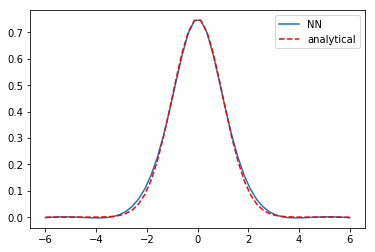## 4 The first excited state

Now, what about the first excited state? This has an eigenvalue of 1.5, and the solution has odd parity. We can naively change the eigenvalue, and hope that the optimizer will find the right new solution. We do that here, and use the old NN params.

params['E'] = 1.6


Now, we run a round of optimization:

params = adam(grad(objective), params,
step_size=0.003, num_iters=5001, callback=callback)

print(params['E'])

Iteration   0 objective [[ 0.09918192]]
Iteration 1000 objective [[ 0.00102333]]
Iteration 2000 objective [[ 0.00100269]]
Iteration 3000 objective [[ 0.00098684]]
Iteration 4000 objective [[ 0.00097425]]
Iteration 5000 objective [[ 0.00096347]]
0.502326347406645



That doesn't work though. The optimizer just pushes the solution back to the known one. Next, we try starting from scratch with the eigenvalue guess.

nnparams = init_random_params(0.1, layer_sizes=[1, 8, 1])

params = {'nn': nnparams, 'E': 1.6}

step_size=0.003, num_iters=5001, callback=callback)

print(params['E'])

Iteration   0 objective [[ 2.08318762]]
Iteration 1000 objective [[ 0.02358685]]
Iteration 2000 objective [[ 0.00726497]]
Iteration 3000 objective [[ 0.00336433]]
Iteration 4000 objective [[ 0.00229851]]
Iteration 5000 objective [[ 0.00190942]]
0.5066213334684926



That also doesn't work. We are going to have to steer this. The idea is pre-train the neural network to have the basic shape and symmetry we want, and then use that as the input for the objective function. The first excited state has odd parity, and here is a guess of that shape. This is a pretty ugly hacked up version that only roughly has the right shape. I am counting on the NN smoothing out the discontinuities.

xm = np.linspace(-6, 6)[:, None]
ym = -0.5 * ((-1 * (xm + 1.5)**2) + 1.5) * (xm < 0) * (xm > -3)
yp = -0.5 * ((1 * (xm - 1.5)**2 ) - 1.5) * (xm > 0) * (xm < 3)

plt.plot(xm, (ym + yp))
plt.plot(x, (1/np.pi)**0.25 * np.sqrt(2) * x * np.exp(-x**2 / 2), 'r--', label='analytical')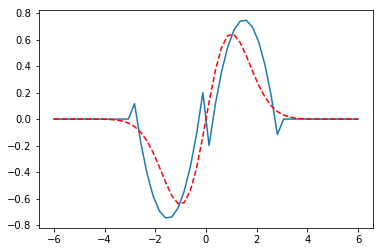Now we pretrain a bit.

def pretrain(params, step):
nnparams = params['nn']
errs = psi(nnparams, xm) - (ym + yp)
return np.mean(errs**2)

step_size=0.003, num_iters=501, callback=callback)

Iteration   0 objective [[ 1.09283695]]



Here is the new initial guess we are going to use. You can see that indeed a lot of smoothing has occurred.

plt.plot(xm, ym + yp, xm, psi(params['nn'], xm))That has the right shape now. So we go back to the original objective function.

params = adam(grad(objective), params,
step_size=0.001, num_iters=5001, callback=callback)

print(params['E'])

Iteration   0 objective [[ 0.00370029]]
Iteration 1000 objective [[ 0.00358193]]
Iteration 2000 objective [[ 0.00345137]]
Iteration 3000 objective [[ 0.00333]]
Iteration 4000 objective [[ 0.0032198]]
Iteration 5000 objective [[ 0.00311844]]
1.5065724128094344



I ran that optimization block many times. The loss is still decreasing, but slowly. More importantly, the eigenvalue is converging to 1.5, which is the known analytical value, and the solution is converging to the known solution.

x = np.linspace(-6, 6)[:, None]
y = psi(params['nn'], x)

plt.plot(x, y, label='NN')
plt.plot(x, (1/np.pi)**0.25 * np.sqrt(2) * x * np.exp(-x**2 / 2), 'r--', label='analytical')
plt.legend()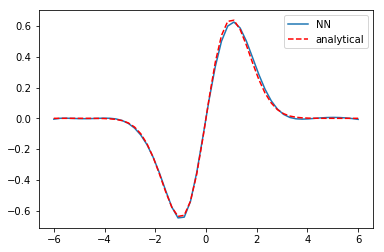We can confirm the normalization is reasonable:

# check the normalization
print(np.trapz(y.T * y.T, x.T))

[ 0.99781886]



## 5 Summary

This is another example of using autograd to solve an eigenvalue differential equation. Some of these solutions required tens of thousands of iterations of training. The groundstate wavefunction was very easy to get. The first excited state, on the other hand, took some active steering. This is very much like how an initial guess can change which solution a nonlinear optimization (which this is) finds.

There are other ways to solve this particular problem. What I think is interesting about this is the possibility to solve harder problems, e.g. not just a harmonic potential, but a more complex one. You could pretrain a network on the harmonic solution, and then use it as the initial guess for the harder problem (which has no analytical solution).